# Help with Statics -- Moment of a Couple

## Homework Statement

This is the problem I'm having problems with.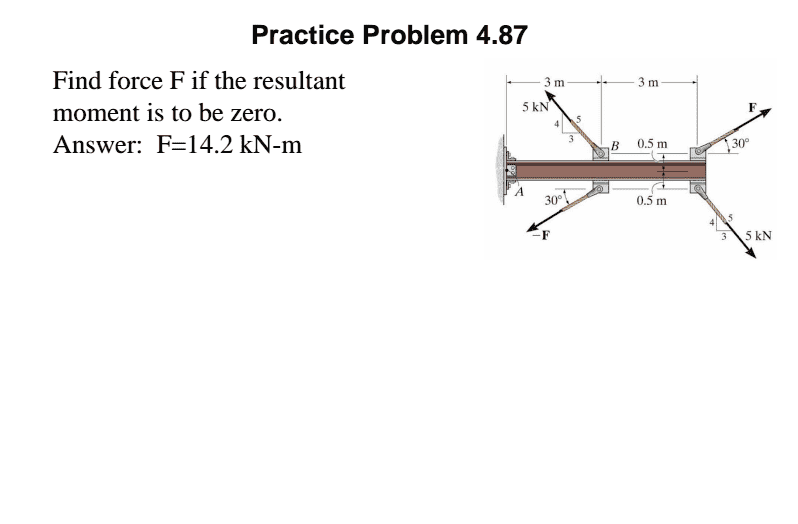M=F x r

## The Attempt at a Solution

Let me explain what I've been doing with the practice problems before it, because I fear I may have the wrong understanding.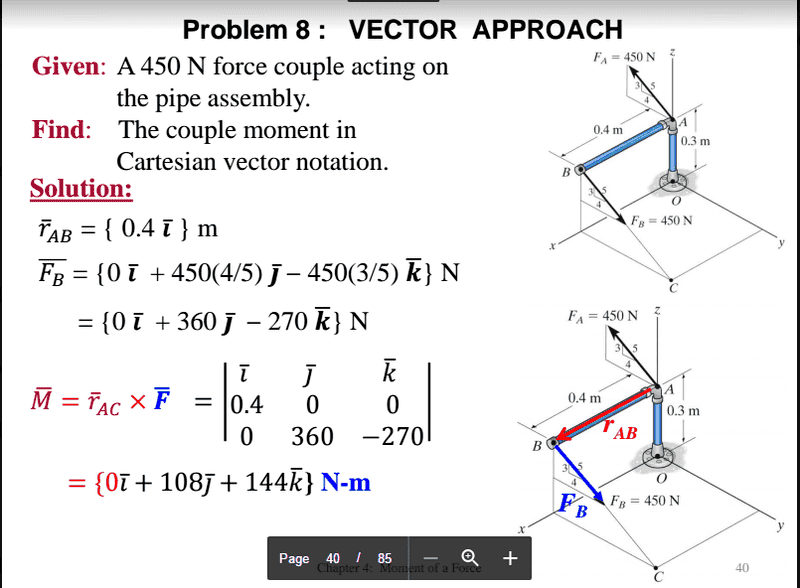For this exercise I just found the perpendicular distance between the couple vectores and put the vectors in cartersian form, then I used the vector product. I know the solution is right there but this is what I did when doing it on my own and got the same result.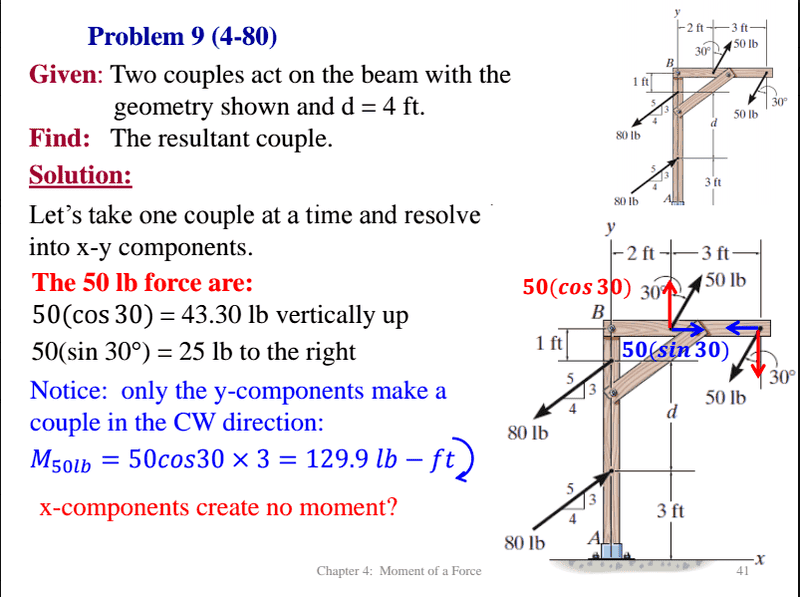I thught I had managed to do this exercise fairly easily but now its confusing me because I think it is similar to the one I'm having probles with but when I try to apply similar steps I'm not getting the correct result. For this one again I found the perpendicular distances between the couple vectors and then multiplied them to get the moment. I thought I had understood why only some components made couples but now I'm confused with the x components of the 50lb force. I thought that since it was in the same direction as the perpendiclar distance that they didn't make a couple but then I noticed that there's a 1ft perpendicular distance. Perhanps it's because the distance isn't BETWEEN THEM?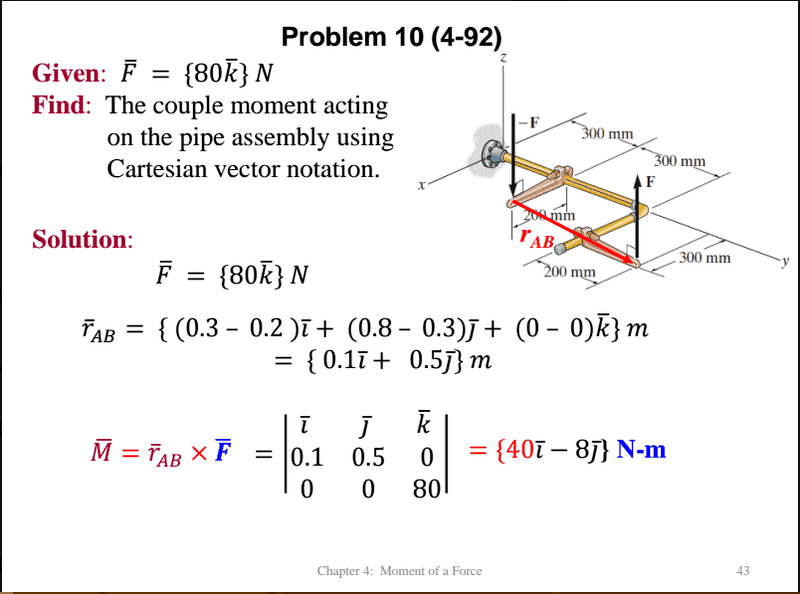Again I was able to do this one easy peasy by finding the distance between the vectors and using vector product.Now back to this one. I separated each force into its components. I THOUGHT the perpendicular distanceof the 5kN forces was 3m and the perp distance of F was 1, but that didn't work out and I'm not getting the result. I saw a solution online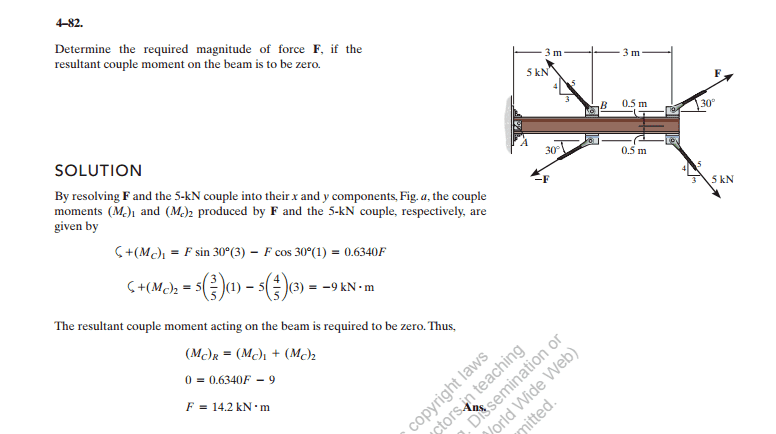I don't understand why they are using both components and why two components of different vectors, say the x components of the F vector and the x component of the 5kN vector, they have the same distance, WHHYYY? Why can't I apply the same logic as before?